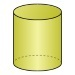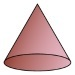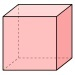##### Notes

Daily Math Practice provides a week's worth of problems ideal for morning work or review. Week 30 reviews standards from previous weeks and supports Common Core Mathematics Standards K.MD.A.1, K.MD.A.2, K.MD.B.3, K.OA.A.4, & K.OA.A.5.

##### Print Instructions

NOTE: Only your test content will print.
To preview this test, click on the File menu and select Print Preview.

See our guide on How To Change Browser Print Settings to customize headers and footers before printing.

# Kindergarten - Daily Math Practice - Week 30 (Kindergarten)

Print Test (Only the test content will print)
 DAILY MATH PRACTICE - WEEK 30 DAY 1 DAY 2 How many more squares are needed to make 10?+ 5 = 10 Add.1 + 1 =Draw a picture that shows the equation. Write the answer.3 + 3 =Mike needs 10 dollars. He has 7 dollars. How many more dollars does Mike need?Answer:dollars Circle the word that describes the temperature of fire.COLD or HOT Circle the one that holds less.How many blocks?16 17 18 Circle the cone.DAILY MATH PRACTICE - WEEK 30 DAY 3 DAY 4 How many more squares are needed to make 10?+ 1 = 10 Subtract.3 - 2 =Cross of the blocks to show the equation. Write the answer.8 - 6 =Emily reads 1 book in school. She reads 3 books at home. How many books does Emily read?Answer:books Circle triangles. Color rectangles.How many triangles?How many rectangles? Circle the one that is colder.Write the missing number.10 += 11 Write the missing number.3 + 4 =+ 2You need to be a HelpTeaching.com member to access free printables.